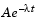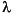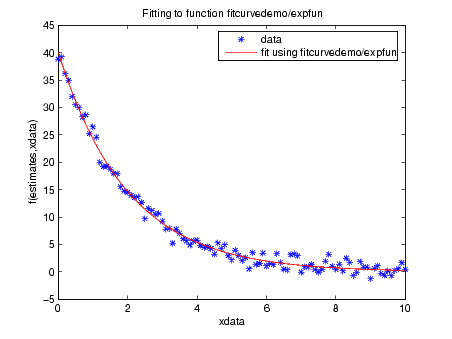MathematicsFitting a Curve to Data

This section gives an example that shows how to fit an exponential function of the formto some data. The example uses the function `fminsearch` to minimize the sum of squares of errors between the data and an exponential functionfor varying parameters A and. This section covers the following topics.

Creating an M-file for the Example

To run the example, first create an M-file that

• Accepts vectors corresponding to the x- and y-coordinates of the data
• Returns the parameters of the exponential function that best fits the data

To do so, copy and paste the following code into an M-file and save it as `fitcurvedemo` in a directory on the MATLAB path.

• ```function [estimates, model] = fitcurvedemo(xdata, ydata)
% Call fminsearch with a random starting point.
start_point = rand(1, 2);
model = @expfun;
estimates = fminsearch(model, start_point);
% expfun accepts curve parameters as inputs, and outputs sse,
% the sum of squares error for A * exp(-lambda * xdata) - ydata,
% and the FittedCurve. FMINSEARCH only needs sse, but we want to
% plot the FittedCurve at the end.
function [sse, FittedCurve] = expfun(params)
A = params(1);
lambda = params(2);
FittedCurve = A .* exp(-lambda * xdata);
ErrorVector = FittedCurve - ydata;
sse = sum(ErrorVector .^ 2);
end
end
```

The M-file calls the function `fminsearch`, which find parameters `A and lambda `that minimize the sum of squares of the differences between the data and the exponential function `A*exp(-lambda*t)`. The nested function `expfun` computes the sum of squares.

Running the Example

To run the example, first create some random data to fit. The following commands create random data that is approximately exponential with parameters `A = 40` and `lambda = .5`.

• ```xdata = (0:.1:10)';
ydata = 40 * exp(-.5 * xdata) + randn(size(xdata));
```

To fit an exponential function to the data, enter

• ```[estimates, model] = fitcurvedemo(xdata,ydata)
```

This returns estimates for the parameters `A` and `lambda`,

• ```estimates =

40.1334    0.5025
```

and a function handle, `model`, to the function that computes the exponential function `A*exp(-lambda*t)`.

Plotting the Results

To plot the fit and the data, enter the following commands.

• ```plot(xdata, ydata, '*')
hold on
[sse, FittedCurve] = model(estimates);
plot(xdata, FittedCurve, 'r')

xlabel('xdata')
ylabel('f(estimates,xdata)')
title(['Fitting to function ', func2str(model)]);
legend('data', ['fit using ', func2str(model)])
hold off
```

The resulting plot displays the data points and the exponential fit.Minimizing Functions of Several Variables Setting Minimization Options© 1994-2005 The MathWorks, Inc.